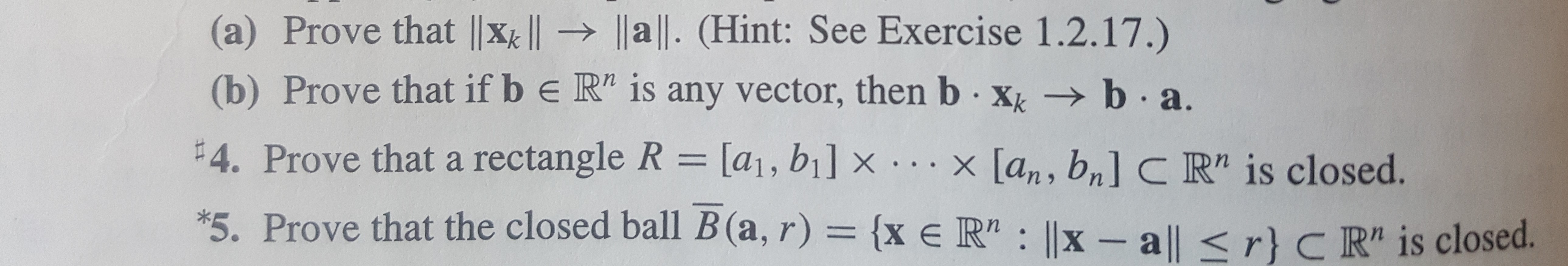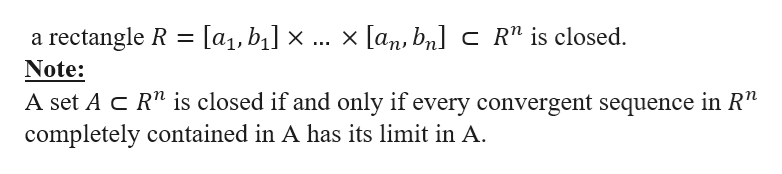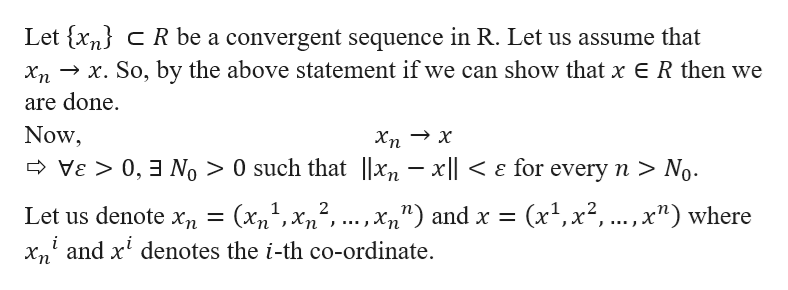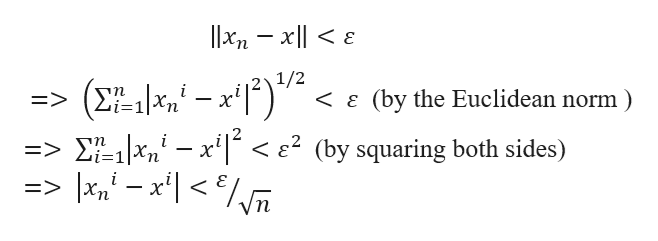# (a) Prove that |x | a ll. (Hint: See Exercise 1.2.17.)(b) Prove that if b e R" is any vector, then b xk b a#4. Prove thata rectangle R = [a1, bi] x ...x [an, bn] C R" is closed.*5. Prove that the closed ball B (a, r) = {x e R" ||x - a|| < r} C R" is closed.

Question
239 views

I need help for number 4. Thanks!help_outlineImage Transcriptionclose(a) Prove that |x | a ll. (Hint: See Exercise 1.2.17.) (b) Prove that if b e R" is any vector, then b xk b a #4. Prove thata rectangle R = [a1, bi] x ...x [an, bn] C R" is closed. *5. Prove that the closed ball B (a, r) = {x e R" ||x - a|| < r} C R" is closed. fullscreen
check_circle

Step 1

We need to show thathelp_outlineImage Transcriptionclosex [an, bn c R" is closed. [a1, b] x a rectangle R Note: A set A C R" is closed if and only if every convergent sequence in R" completely contained in A has its limit in A fullscreen
Step 2

Now,help_outlineImage TranscriptioncloseLet {xn CR be a convergent sequence in R. Let us assume that x. So, by the above statement if we can show that x ER then we are done Now VE 0,3 No > 0 such that |2n - xl No Хn _ - (х,, хв xand x denotes the i-th co-ordinate. 2 (x1,x2, .., x") where ...., xn") and x = Let us denote xn i fullscreen
Step 3

Further,...help_outlineImage Transcriptionclose1/2 Zi=1 nx e2 (by squaring both sides) I* - xl < /vm => => fullscreen

### Want to see the full answer?

See Solution

#### Want to see this answer and more?

Solutions are written by subject experts who are available 24/7. Questions are typically answered within 1 hour.*

See Solution
*Response times may vary by subject and question.
Tagged in

### Math